### Home > CALC > Chapter 11 > Lesson 11.4.1 > Problem11-130

11-130.
1. Multiple Choice: The luminous intensity E of a light bulb, measured in lumens per ft2, varies inversely as the square of the distance s from the bulb. E = 5.2 lumens per ft2 when s = 5 ft for a 100 watt bulb. If you are moving away from a 100 watt bulb at a speed of 2 feet per second and you are 3 feet from the bulb, the luminous intensity is changing at the rate of: Homework Help ✎

1.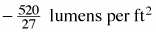2.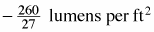3.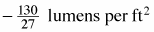4.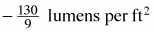5.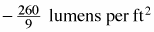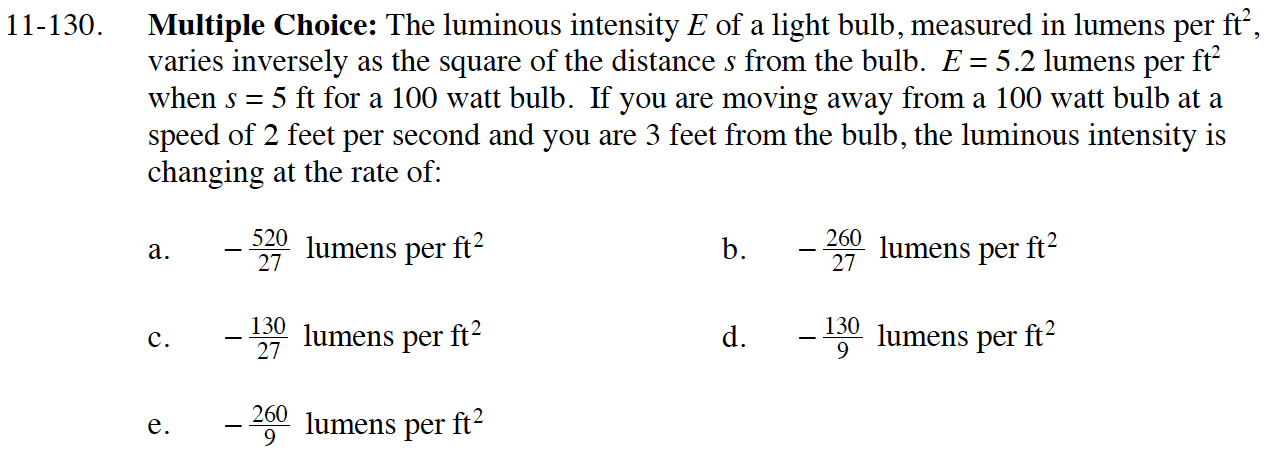$5.2=\frac{k}{5^2}\text{ }k=?$

When s = 3, E = ?

Substitute in the known values and solve for E′.